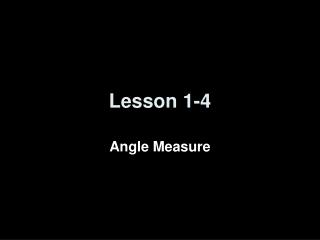Download PresentationLesson 1-4

Loading in 2 Seconds...

# Lesson 1-4 - PowerPoint PPT Presentation

Lesson 1-4. Angle Measure. Lesson Outline. Five-Minute Check Then & Now and Objectives Vocabulary Key Concept Examples Lesson Checkpoints Summary and Homework. Then and Now. You measure line segments (Lesson 1–2). Measure and classify anglesI am the owner, or an agent authorized to act on behalf of the owner, of the copyrighted work described.
Download Presentation## Lesson 1-4

An Image/Link below is provided (as is) to download presentation

Download Policy: Content on the Website is provided to you AS IS for your information and personal use and may not be sold / licensed / shared on other websites without getting consent from its author.While downloading, if for some reason you are not able to download a presentation, the publisher may have deleted the file from their server.

- - - - - - - - - - - - - - - - - - - - - - - - - - E N D - - - - - - - - - - - - - - - - - - - - - - - - - -
Presentation Transcript
1. Lesson 1-4 Angle Measure

2. Lesson Outline • Five-Minute Check • Then & Now and Objectives • Vocabulary • Key Concept • Examples • Lesson Checkpoints • Summary and Homework

3. Then and Now You measure line segments (Lesson 1–2) • Measure and classify angles • Identify and use congruent angles an the bisector of an angle

4. Objectives • Measure and classify angles • Obtuse – m > 90 • Right – m = 90 (the corner of a piece of paper) • Acute – m < 90 • Identify and use congruent angles an the bisector of an angle

5. Vocabulary • Degree – one three hundred and sixtieth of a circle • Ray – part of a line with one end point • Opposite rays – are collinear rays with the same end point (& form a 180 degree angle) Angle is formed by 2 noncollinear rays with a common endpoint (vertex) • Sides – composed of rays • Vertex – is the common endpoint • Interior – area between the two rays that form the angle • Exterior – area not between the two rays that form the angle

6. Vocabulary (cont) Special types of angles: • Right angle – measure equals 90 degrees • Acute angle – measure is less than 90 degrees • Obtuse angle – measure is greater than 90 degrees (but less than 180) • Angle Bisector – a ray that divides an angle into two congruent angles

7. Answer: and or are the sides of 5. Example 1 Name all angles that have B as a vertex. Answer:5, 6, 7, and ABG Name the sides of 5. Write another name for 6. Answer:EBD, FBD, DBF, and DBE are other names for 6.

8. Key Concept • An easy measuring device is the corner of a piece of notebook paper (or computer paper). It is a right angle.

9. Answer: is a right angle. Example 2a Measure TYV and classify it as right, acute, or obtuse. TYV is marked with a right angle symbol, so measuring is not necessary.

10. Use a protractor to find that . Answer: > is an obtuse angle. Example 2b Measure WYT and classify it as right, acute, or obtuse.

11. Example 2c Measure TYU and classify it as right, acute, or obtuse. Use a protractor to find that m TYU = 45° . Answer: Since 45 < 90, then TYU is an acute angle.

12. Example 3 INTERIOR DESIGN Wall stickers of standard shapes are often used to provide a stimulating environment for a young child’s room. A five-pointed star sticker is shown with vertices labeled. Find mGBH and mHCI if GBH  HCI, mGBH = 2x + 5, and mHCI =3x – 10. Step 1 Solve for x. GBH  HCI Given

13. Example 3 cont mGBH = mHCI Definition of congruent angles 2x + 5 = 3x – 10 Substitution 2x + 15 = 3x Add 10 to each side. 15 = x Subtract 2x from each side. Step 2 Use the value of x to find the measure of either angle. mGBH = 2x + 5Given = 2(15) + 5 Substitution, x = 15 = 30 + 5 = 35 Simplify. Answer: mGBH = 35, mHCI = 35

14. Lesson Checkpoints

15. Summary & Homework • Summary: • Angles are named by 3 points with the vertex in the middle (or by vertex letter if no confusion) • Angles are classified as acute, right, or obtuse according to their measure • An angle bisector is a ray that divides an angle into two congruent angles (halves) • Ray is equidistance from the sides of the angle • Homework: • pg 41-3: 30-35, 39-42